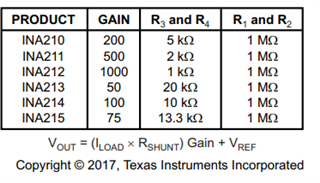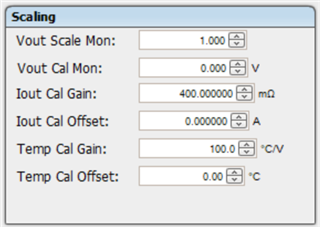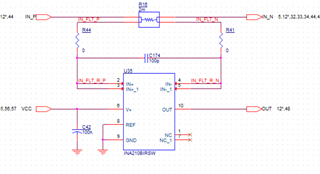If you have a related question, please click the "Ask a related question" button in the top right corner. The newly created question will be automatically linked to this question.

# INA210: iNA210 question

Part Number: INA210
Other Parts Discussed in Thread: UCD90320,

1. Do you have equation for calculating Rshunt value ?

2. We are using UCD90320 Amon pin for ADC converting. Therefore, Ｉneed to choose Iout Cal Gain and Iout Cal Offset in fusion desgin tool, see figure 1.

Can you teach me how to select the gain and offset ?

3. Can you help to review the schematic in figure 2?

4.  According to equatoin and gain= 200 shown beIow, I will get 0.16V at out pin when the load is 0.4A in figure 2, right?Figure 1Figure 2

1. VCC=3.3V

2. Output = ?

3. Rshunt = 2m ohm• Hello Mike,

See my replies below.

1. Do you have equation for calculating Rshunt value ?

This is normally a tradeoff with voltage drop and power loss.  You normally get more signal the more you increase the value of your Rshunt but you lose more power across your Rshunt.  Normally the larger your Rshunt the lower gain you would need.  You must also look at offset and swing low to determine the lowest value you could measure.  See https://training.ti.com/ti-precision-labs-current-sense-amplifiers Section 2.2 to look at this in more detail.

2. We are using UCD90320 Amon pin for ADC converting. Therefore, Ｉneed to choose Iout Cal Gain and Iout Cal Offset in fusion desgin tool, see figure 1.

Can you teach me how to select the gain and offset ?

Please ask the UCD90320 team as this is the tool they design and support.  I will forward this one to them.

3. Can you help to review the schematic in figure 2?

I reviewed and this looks fine.  Max current~Vsupply/Gain/Rshunt ~ Max current  5V/200/0.002= 12.5A.  This is assuming the 2mΩ resistance can handle the power.

4.  According to equatoin and gain= 200 shown beIow, I will get 0.16V at out pin when the load is 0.4A in figure 2, right?  This is correct.

• Hi

The Gain and offset shall be based on the INA and sensing resistor used in your system.

UCD90320 only monitors voltage. it converts the voltage to current based on the gain and offset.

For example, if you have a voltage reading at 0.5V and the expect current is 5A. you shall set the gain to 100mohms.

Regards

Yihe

• hi

I think I understand what you mean for setting Iout Cal Gain

I give another example. 1.2V power rail with max current 1.4A. The reading from INA210 is 0.56V. It means Iout Cal Gain shall be

400m ohmright?  0.56V / 1.4A = 0.4 ohm = 400m ohm

Mike Hsu

• Hi

If 0.56V is the read when reaching 1.4A, you are right

Regards

Yihe